Search

Is the Inverse a Function? (page 2 of 7)

Sections: Definition / Inverting a graph, Is the inverse a function?, Finding inverses, Proving inverses

Now suppose your function is { (1, 2), (2, 1), (3, 4), (5, 1) }. The inverse of this function is { (2, 1), (1, 2), (4, 3), (1, 5) }. This inverse has two points, (1, 2) and (1, 5), that share a common x-value but have different y-values. This means that the inverse is NOT a function.

 Graphically, the original function looks like this: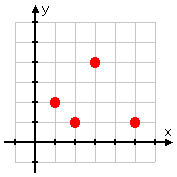You can find the inverse algebraically, by flipping the x- and y-coordinates, or graphically, by drawing the line y = x...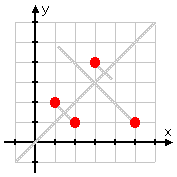Copyright © Elizabeth Stapel 2000-2011 All Rights Reserved ...and reflecting all the points across it: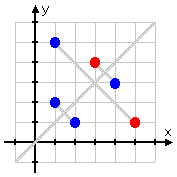Note that it's perfectly okay for the inverse to "overwrite" the original function's points! The points "(2, 1) and (1, 2)" of the inverse overwrote the points "(1, 2) and (2, 1)" of the original function, which is why the graph is "missing" a red dot.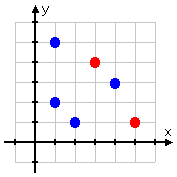But you can see that the inverse is not a function: there are two points sharing an x-value.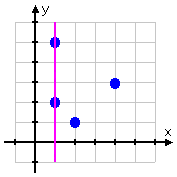There is a quick way to tell, before going to the trouble of finding the inverse, whether the inverse will also be a function. You've seen that you sort of "flip" the original function over the line y = x to get the inverse. Using this fact, someone noticed that you can also "flip over" the Vertical Line Test to get the Horizontal Line Test. As you can see, you can draw a horizontal line through two of the points in the original function: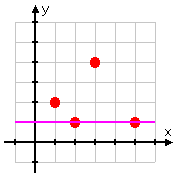Since the original function had two points that shared the same Y-VALUE, then the inverse of the original function will not be a function. This means, for instance, that no parabola (quadratic function) will have an inverse that is also a function.

In general, if the graph does not pass the Horizontal Line Test, then the graphed function's inverse will not itself be a function; if the list of points contains two or more points having the same y-coordinate, then the listing of points for the inverse will not be a function. So when you're asked "Will the inverse be a function?", if you're given a graph, draw a horizontal line; if you're given a list of points, compare the y-coordinates.

<< Previous  Top  |  1 | 2 | 3 | 4 | 5 | 6 | 7  |  Return to Index  Next >>

 Cite this article as: Stapel, Elizabeth. "Is the Inverse a Function?." Purplemath. Available from     https://www.purplemath.com/modules/invrsfcn2.htm. Accessed [Date] [Month] 2016

Study Skills Survey

Tutoring from Purplemath
Find a local math tutor

 Copyright © 2021  Elizabeth Stapel   |   About   |   Terms of Use   |   Linking   |   Site Licensing Contact Us